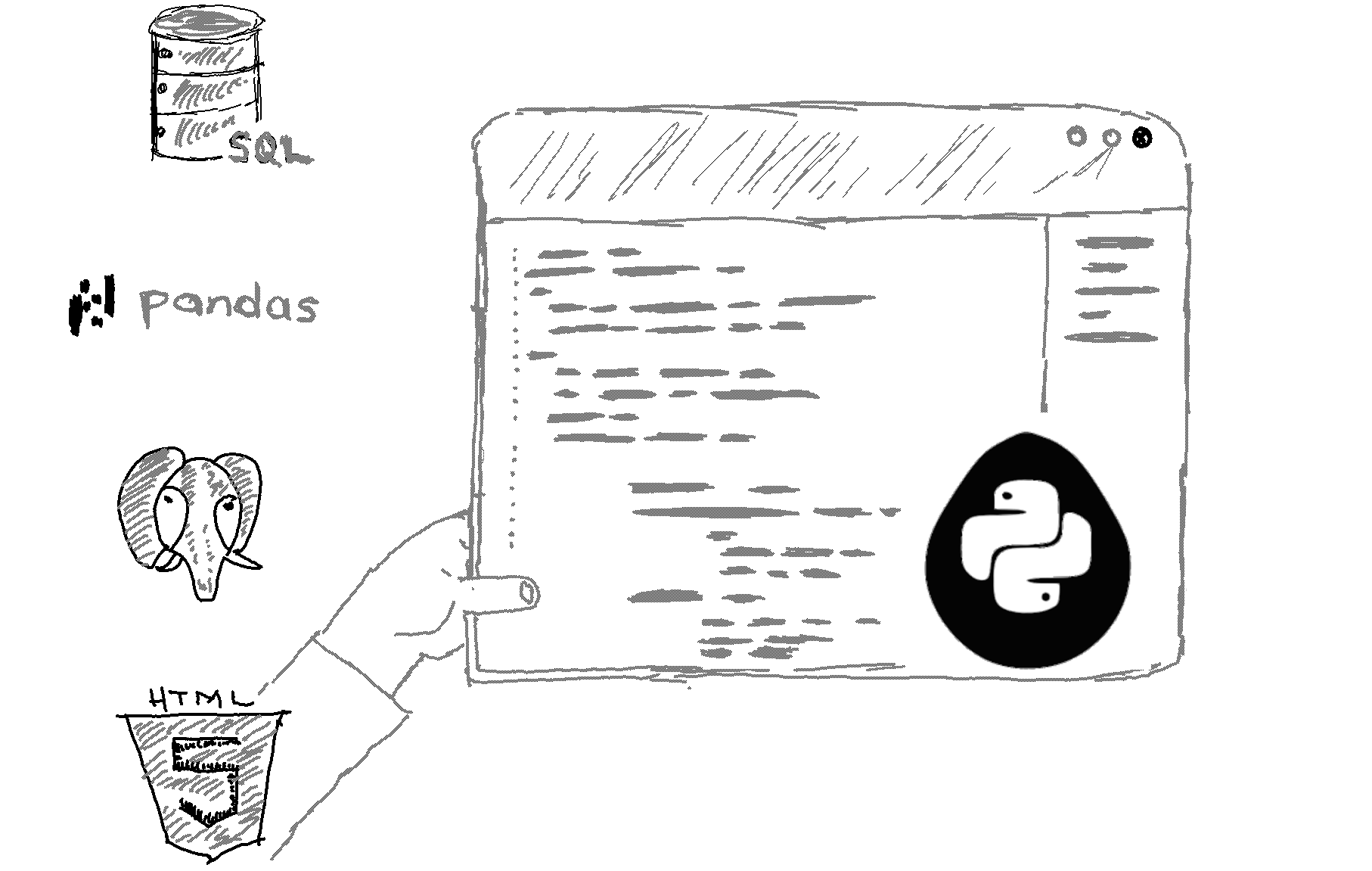# Here is how to rename column names with Pandas in Python.

```import pandas as pd

# create a sample DataFrame
df = pd.DataFrame({
"A": [1, 2, 3],
"B": [4, 5, 6],
"C": [7, 8, 9]
})

# rename the columns of the DataFrame
df = df.rename(columns={"A": "X", "B": "Y", "C": "Z"})

# the DataFrame now has new column names
print(df) ```

Output
```   X  Y  Z
0  1  4  7
1  2  5  8
2  3  6  9 ```

Explanation

To rename the columns of a DataFrame in Pandas, you can use the DataFrame.rename() method. This method allows you to specify a new name for one or more columns by providing a mapping of old column names to new column names.

In the example above, the df.rename() method takes a columns parameter that is a dictionary mapping the old column names (the keys of the dictionary) to the new column names (the values of the dictionary). The resulting DataFrame will have the new column names.

You can also use the inplace parameter of the rename() method to modify the DataFrame in place, without assigning the result to a new variable. Here is an example:

```import pandas as pd

# create a sample DataFrame
df = pd.DataFrame({
"A": [1, 2, 3],
"B": [4, 5, 6],
"C": [7, 8, 9]
})

# rename the columns of the DataFrame in place
df.rename(columns={"A": "X", "B": "Y", "C": "Z"}, inplace=True)

# the DataFrame now has new column names
print(df)```
*Note that the rename() method only changes the column names of the DataFrame. It does not change the data in the DataFrame.

Related HowTos
##### Recommended Course

Learn Flask development and learn to build cool apps with our premium Python course on Udemy.